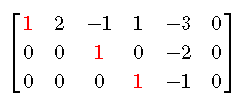# Free and Basic Variables of a Matrix - Examples with Solutions

## Free and Basic Variables of a Matrix Representing a System of Equations

Definition For a system of equations in row echelon form , which of course may be represented by the augmented matrix, a variable whose coefficient is a leading 1 ( pivot ) is called a basic variable and a varibale without pivot is called a free variable. Let us consider the following system of equations in row echelon formThe augmented matrix in row echelon of the above system is as followsAccording fo the above definition
and ${x}_{4}$ are the basic variables and ${x}_{2}$ and ${x}_{5}$ are the free variables.
When we solve the above system, we express the basic variables in terms of the free variables
The third equation in the system gives
${x}_{4}={x}_{5}$
The second equation gives
${x}_{3}=2{x}_{5}$
The first equation gives
${x}_{1}=-2{x}_{2}+{x}_{3}-{x}_{4}+3{x}_{5}$
Substitute the basic variables on the right
${x}_{1}=-2{x}_{2}+2{x}_{5}-{x}_{5}+3{x}_{5}$
Simplify
${x}_{1}=-2{x}_{2}+4{x}_{5}$
The basic variables are written in terms of the free variables as
${x}_{1}=-2{x}_{2}+4{x}_{5}$
${x}_{3}=2{x}_{5}$
${x}_{4}={x}_{5}$
where ${x}_{2}$ and ${x}_{5}$ can be any real numbers hence their names as "free variables".
Definition The use of free variables helps us to write an explicit formula for the solutions of our system.

## Questions with Solution

For each of the following augmented matrices in row echelon form, which are basic variables and which are free variables?

1. $\left[\begin{array}{ccccc}1& 4& 3& 0& 0\\ 0& 0& 1& -3& 1\\ 0& 0& 0& 0& 0\end{array}\right]$

2. $\left[\begin{array}{ccc}1& 0& 0\\ 0& 1& 0\end{array}\right]$

3. $\left[\begin{array}{cccccc}1& 2& 0& -1& -2& 0\\ 0& 1& 1& 0& 3& 0\\ 0& 0& 0& 1& 1& 0\\ 0& 0& 0& 0& 0& 0\end{array}\right]$

4. $\left[\begin{array}{cccc}1& 2& 0& 0\\ 0& 0& 1& 0\\ 0& 0& 0& 0\end{array}\right]$

## Solutions to the Above Questions

Being augmented matrices, the number of variables is equal to the number of columns of the given matrix -1.
For examples, for a matrix of 5 columns, the number of variables is 5 - 1 = 4, named as ${x}_{1}$, ${x}_{2}$, ${x}_{3}$ and ${x}_{4}$.

1. Matrix 1 is has two pivots and 4 variables.
The first pivot at row 1 column 1; hence ${x}_{1}$ is a basic variable.
The second pivot is at row 2 column 3; hence ${x}_{3}$ is also a basic variable.
The remaining variables: ${x}_{2}$ and ${x}_{4}$ are free variables.

2. Matrix 2 is has two Pivots and 2 variables.
The first pivot is at row 1 column 1; hence hence ${x}_{1}$ is a basic variable.
The second pivot is at row 2 column 2; hence ${x}_{2}$ is a basic variable.
There are no free variables.

3. Matrix 3 has 3 pivots and 5 variables.
The first pivot at row 1 column 1; hence ${x}_{1}$ is a basic variable.
The second pivot is at row 2 column 2; hence ${x}_{2}$ is a basic variable.
The third pivot is at row 3 column 4; hence ${x}_{4}$ is a basic variable.
The remaining variables: ${x}_{3}$ and ${x}_{5}$ are free variables.

4. Matrix 4 has two Pivots and 3 variables.
The first pivot is at row 1 column 1; hence hence ${x}_{1}$ is a basic variable.
The second pivot is at row 2 column 3; hence ${x}_{3}$ is a basic variable.
The remaining variables: ${x}_{2}$ is a free variables.